# 基本元器件 - 晶体三级管

## 区分极性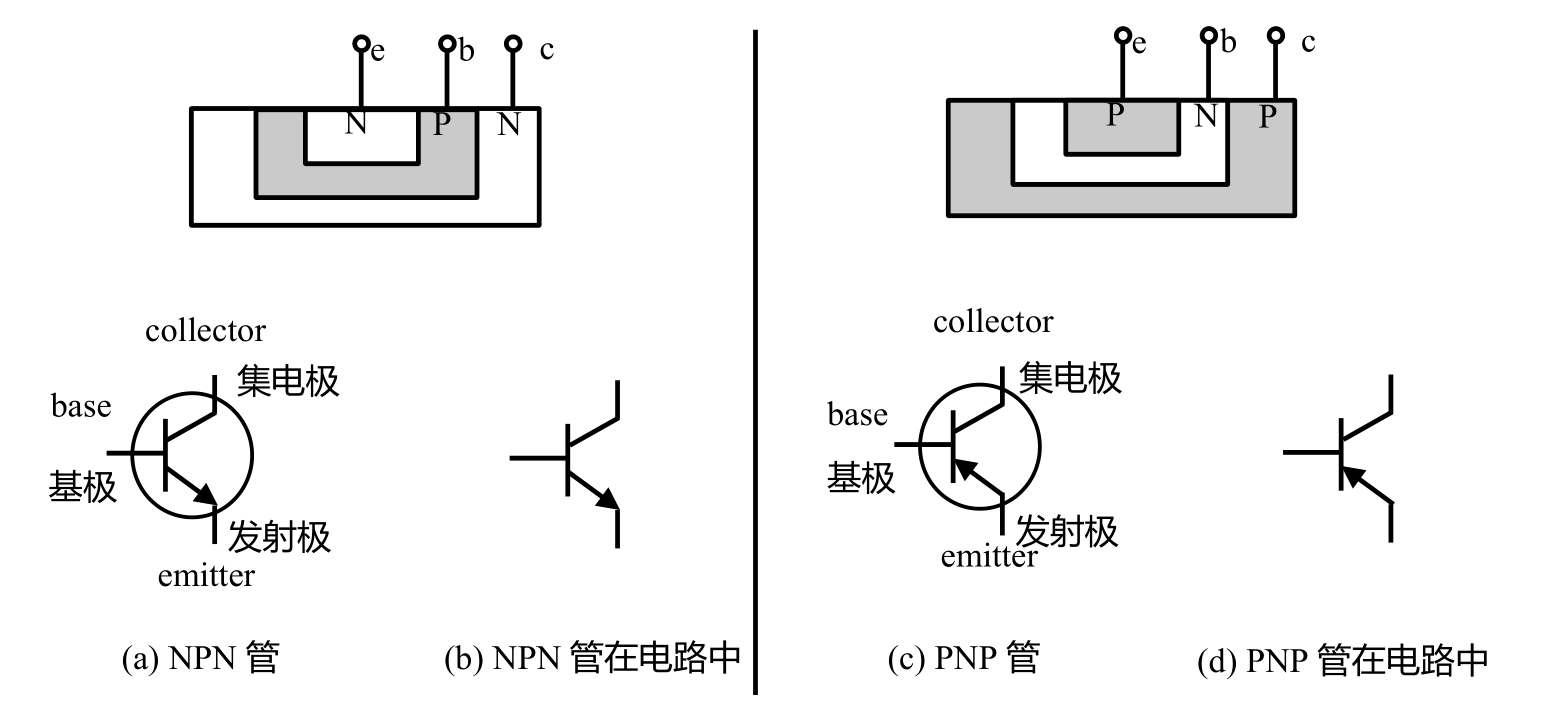## 不同封装引脚辨认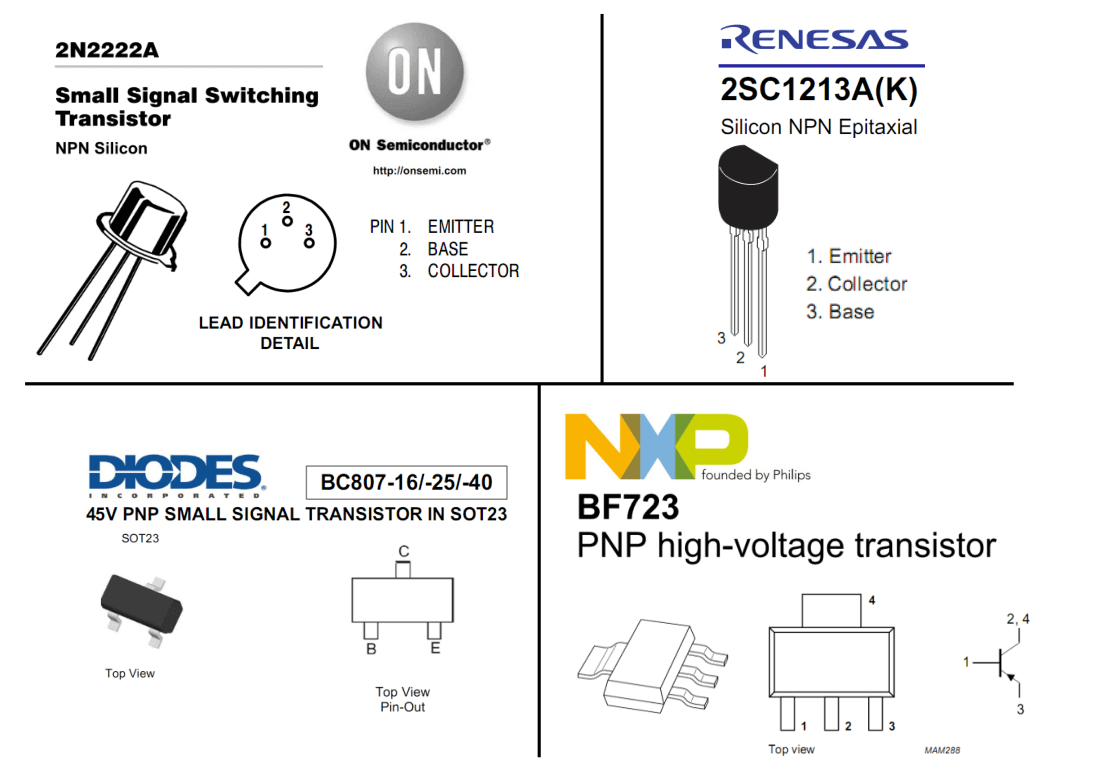## 基本电流关系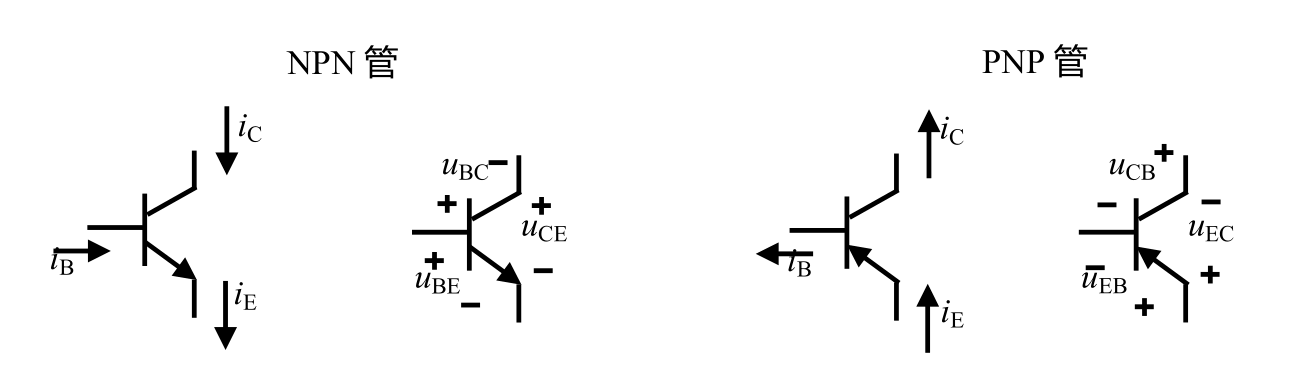• NPN：发射级流出，所以基极和集电极都是流入。
• PNP：发射级流入，所以基极和集电极都是流出。

1. 满足基尔霍夫电流定律 $$i_B + i_C = i_E$$
2. 处于放大状态下，集电极电流只受控于基极电流（$$i_C = \beta i_B$$），与集电极发射极间的电压无关。
3. 基极与发射极导通时，分压值 $$U_{BE}$$ 约为 0.7V

## 输出伏安特性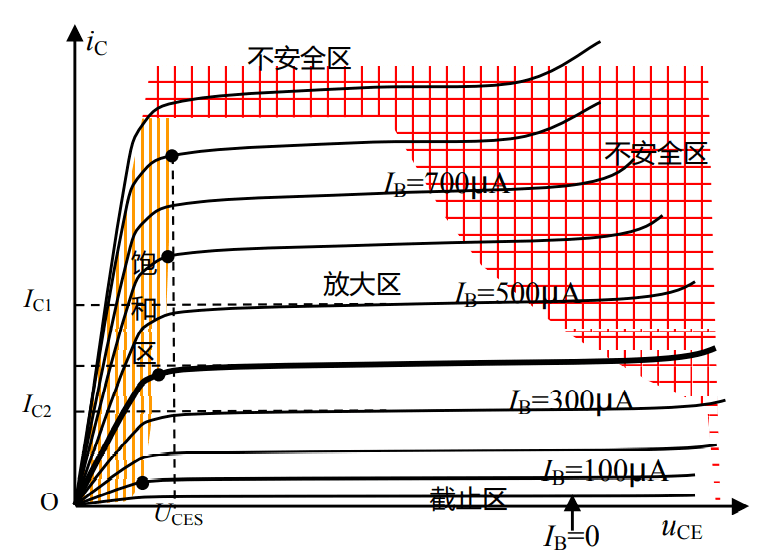• 放大区：在此区域内，晶体管的 $$i_C$$ 几乎不随 $$u_{CE}$$ 变化，近似满足 $$i_C = \beta i_B$$
• 饱和区：在此区域内，晶体管的 $$i_C$$ 随着 $$u_{CE}$$ 增大而增大。一般认为当 $$u_{CE}$$ 小于饱和压降 $$U_{CES}$$（一般为 0.3 V）时，晶体管工作在饱和区。
• 截止区：即 $$I_B = 0$$ 的那根曲线。但此时 $$i_C$$ 并不为 0，因为存在与 $$u_{CE}$$ 相关的漏电流。截止区代表晶体管处于几乎没有任何电流进出的状态，近似于完全关闭。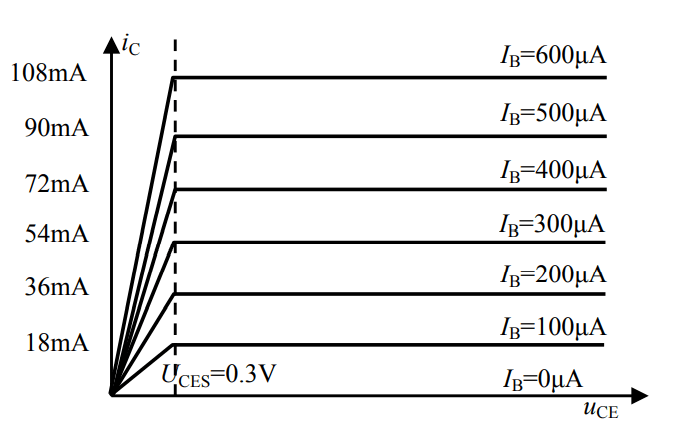• 放大区：满足 $$i_C = \beta i_B$$，与 $$u_{CE}$$ 无关。
• 饱和区$$i_C$$ 随着 $$u_{CE}$$ 增大而增大，近似为线性。
• $$U_{CES}$$ 垂直线：饱和区与放大区的分界线。

## 晶体管的工作状态

• 截止状态
• 指基极未产生明显电流（$$I_{BQ}$$ 非常小导致 $$I_{CQ}$$ 也很小），集电极与发射极之间相当于开路。
• $$I_{BQ} = 0, I_{CQ} = 0, I_{EQ} = I_{BQ}+I_{CQ}=0$$。发射结零偏 / 反偏、集电结反偏。
• 放大状态
• 指晶体管处于 $$I_{BQ}$$ 合适，且满足 $$I_{CQ} = \beta I_{BQ},I_{EQ} = (1+ \beta)I_{BQ}, I_{BQ} = \frac{V_{CC}-U_{BE}}{R_B}$$
• 发射结正偏、集电结反偏。
• 这是模电最常用的状态。
• 饱和状态
• $$I_{CQ} < \beta I_{BQ}$$，但还是随 $$U_{CEQ}$$ 变化。$$I_{BQ}$$$$I_{CQ}$$ 都很大，$$I_{CQ}$$ 已经不完全受 $$I_{BQ}$$ 控制，且 $$U_{CEQ}$$ 所占的电压很小。
• 只要 $$U_{CEQ} < U_{CES}$$，就进入饱和状态。此时，再增加 $$I_{BQ}$$$$I_{CQ}$$ 也几乎不再增加。
• 发射结正偏、集电结正偏。
• 在模电中应该避免进入饱和状态，而在数电中则期望进入饱和或截至状态。
• 倒置状态
• 集电极和发射极接反了。虽然也不是不能用，但是会造成 $$\beta$$ 下降严重。
• 饱和状态就好比水龙头打开了，但水箱里没水，此时就是有多少水来多少水。
• 发射结反偏，集电结正偏。

## 三极管的主要参数

• 电流放大系数 $$\beta$$：一般为 10-100 倍，但在应用中取 30-80 倍为宜（太小放大不明显，太大工作不稳定）。
• 集电极最大允许电流 $$I_{CM}$$：超过可能导致烧坏。
• 集电极最大允许功耗 $$P_{CM}$$
• 集电极发射极间反向击穿电压 $$V_{CEO}$$

## 估算法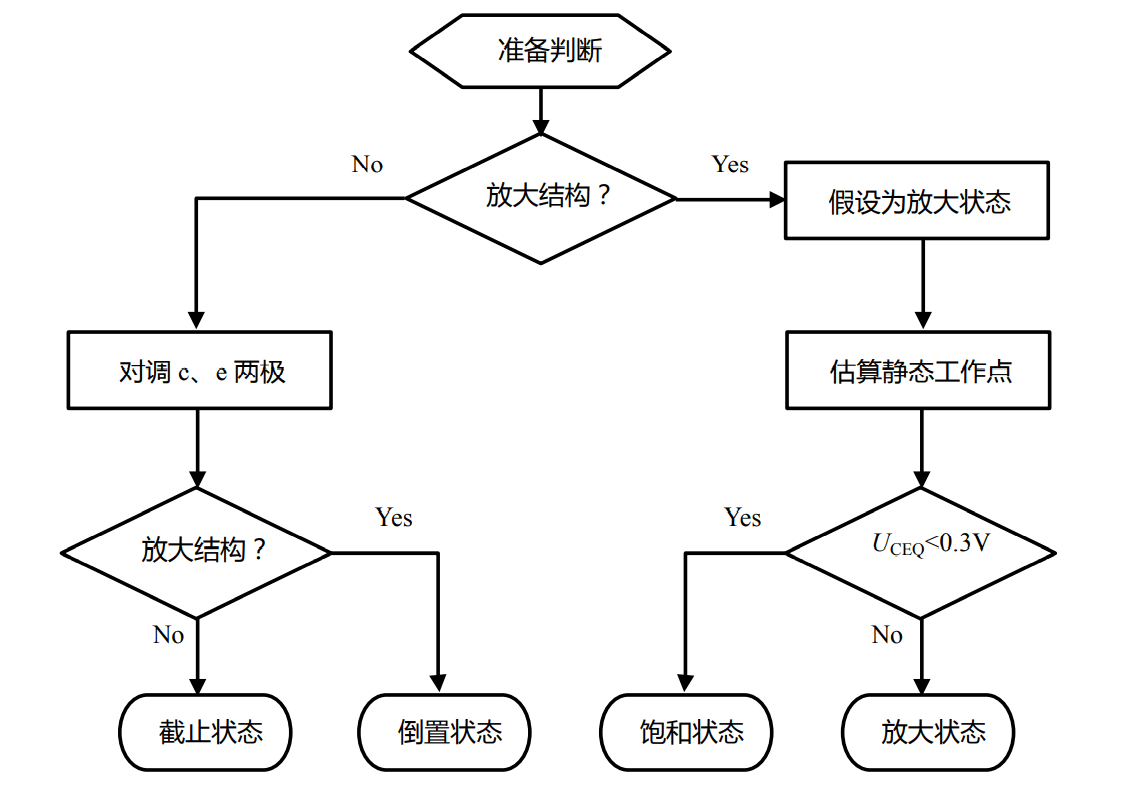1. 根据 $$U_{BEQ} = 0.7 V$$，算出 $$I_{BQ}$$
2. 假设处于放大状态，即 $$I_{CQ} = \beta I_{BQ}$$，求解出 $$U_{CEQ}$$
3. 此时如果 $$U_{CEQ} >= 0.3 V$$，则假设成立，晶体管处于放大状态，$$I_{CQ}$$$$U_{CEQ}$$ 为所求。
4. 如果 $$U_{CEQ} < 0.3 V$$，则假设不成立，晶体管处于饱和状态。

## 基本放大电路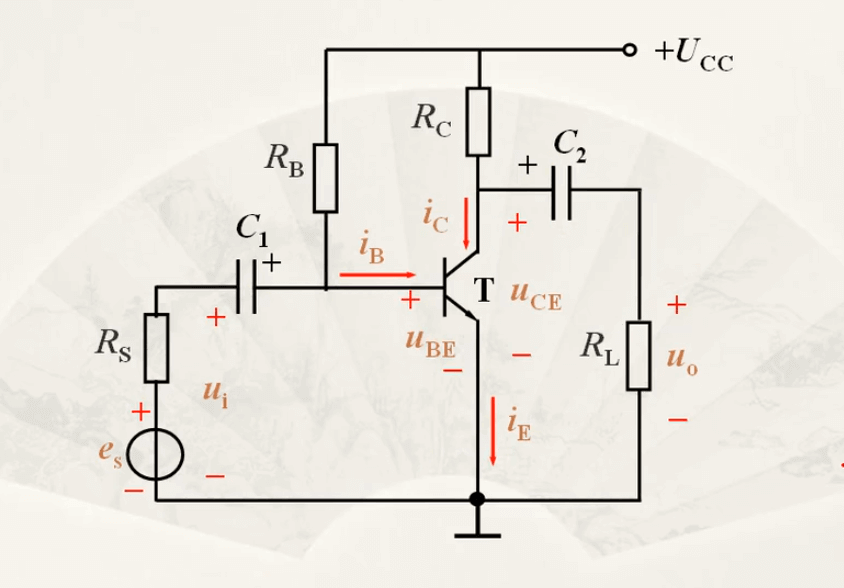• $$C_1$$/$$C_2$$：隔直通交。排除 $$U_{CC}$$ 的影响。取值几微法到几十微法。
• $$U_{CC}$$：为电路功能；提供合适的静态工作点。
• $$R_B$$：提供合适的 $$I_B$$，取值一般为几十欧到几百千欧。
• $$R_C$$：取值几千欧到几十千欧。

• 总基极电压 $$U_{BE} = U_{BEQ}+u_i$$
• 总基极电流 $$i_B=I_{BQ}+i_b$$
• 总集电极电流 $$i_C=I_{CQ}+i_c$$
• 总的 $$u_CE=V_{CC}-{i_C}{R_C}=V_{CC}-(I_{CQ}+i_c)R=U_{CEQ}+({-i_C}{R_C})$$